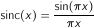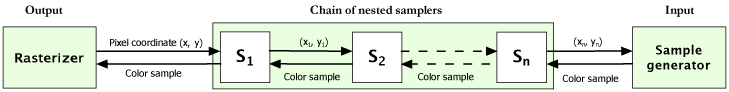# Sampling and Rasterization

## Sampling

Sampling is a very important concept within digital image processing and image analysis. Sampling is a process where color samples are acquired given their logical coordinates in the (x, y) coordinate space. Graphics32 provides a special class called TCustomSampler, that provides the necessary mechanism for implementing different sampling techniques. A sampler can be conceived as a scalar function f(x, y) that returns a color sample given a logical coordinate (x, y). A sample may be created synthetically (this is a common technique within ray-tracing, fractal rendering and pattern generation). It may also be acquired from some input hardware device. Another very common method for acquiring samples is resampling.

## Resampling

Resampling is the process of reconstructing samples from a discrete input signal. The idea can also be extended from the 1D case to 2D. In the 2D case we can think of the bitmap as our signal. We have a number of pixels, aligned on a rectangular square grid. Hence we only know the actual color values at a number of discrete coordinates. In order to determine the color value of a sample at an arbitrary coordinate in a continuous image space, we need to perform interpolation for reconstructing this sample.

Descendants of TCustomResampler implement various algorithms for performing resampling and sample acquisition. A general algorithm reconstructing samples is to perform convolution in a local neighborhood of the actual sample coordinate. This method is used in TKernelResampler, where a convolution filter is specified by the TKernelSampler.Kernel property.

Graphics32 includes a class called TCustomKernel which is used as an ancestor class for various convolution kernels. For high quality resampling, one should consider using a kernel that approximates the ideal low-pass filter. The ideal low-pass filter is often referred to as a sinc filter. It can be described by the formulaSince this function has infinite extent, it is not practical for using as a convolution kernel (because of the computational overhead). TWindowedSincKernel is a base class for kernels that use the sinc function together with a window function (also known as tapering function or apodization function). This way the kernel can be constrained to a certain width and reduce the amount of computations.

For further details about resampling, see Resamplers_Ex example project.

## Rasterization

By rasterizing an image, we collect samples for each pixel of an output bitmap. The rasterizer is responsible for the order in which output pixels are sampled and how the destination bitmap is updated. A rasterizer class is derived from TRasterizer, by overriding the protected DoRasterize method.

Instances of TRasterizer needs to be associated with a sampler and an output destination bitmap. Some rasterization schemes, such as swizzling, may improve cache-performance for certain applications, since samples are collected in a local neighborhood rather than row by row. Rasterizers can also provide various transition effects for creating transitions between bitmaps.

Graphics32 includes the following rasterizers:

## Nested sampling

If the input of one sampler is the output from another, then we have a nested sampler. Nested samplers are derived from the class TNestedSampler.

By nesting samplers, it is possible to create a chain of nested samplers between the sampler that generates the actual sample and the rasterizer. This mechanism is illustrated in the below image.There are many different useful applications for nested samplers. A sampler may be associated with a transformation. This will transform the input coordinate that is passed to the sampler at the next level.

It is possible to collect more than one sample in a local neighborhood of the pixel coordinate of the output pixel. This permits the use of techniques such as super sampling, where several samples are collected in order to estimate the color of the area covered by a pixel in the destination bitmap. If super sampling is not performed, it may cause jagginess and aliasing artifacts in the output image. However, this also depends on what kind of reconstruction method is used if samples are resampled.

Another important class of nested samplers is kernel samplers. Kernel samplers compute an output sample from several subsamples in a local region of the input coordinate. Each subsample is combined with a kernel value (contained within a TIntegerMap object). A class-specific kernel operation is used to update a buffer for each collected sample. This permits a very simplistic implementation of convolution and morphological operations.

The following is a list of the different nested samplers that are included in Graphics32.